# A Tutorial on Identification of Nonlinear ARX and Hammerstein-Wiener Models

This example shows identification of single-input-single-output (SISO) nonlinear black box models using measured input-output data. The example uses measured data from a two-tank system to explore various model structures and identification choices.

### The Input-Output Data Set

In this example, the data variables stored in the `twotankdata.mat` file will be used. It contains 3000 input-output data samples of a two-tank system generated using a sample time of 0.2 seconds. The input u(t) is the voltage [V] applied to a pump, which generates an inflow to the upper tank. A rather small hole at the bottom of this upper tank yields an outflow that goes into the lower tank, and the output y(t) of the two tank system is then the liquid level [m] of the lower tank. We create an IDDATA object `z` to encapsulate the loaded data:

This data set is also used in the nonlinear grey box example "Two Tank System: C MEX-File Modeling of Time-Continuous SISO System" where physical equations describing the system are assumed. This example is about black box models, thus no knowledge of the actual physical laws is assumed.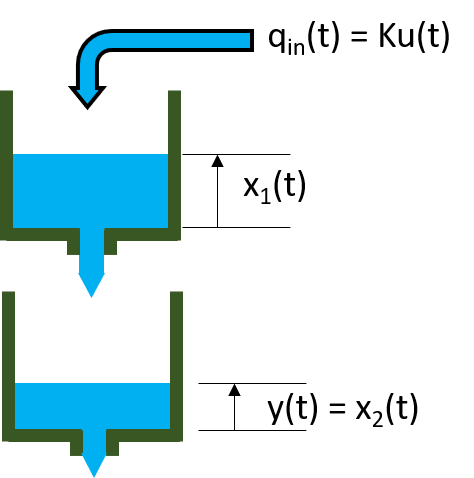```load twotankdata z = iddata(y, u, 0.2, 'Name', 'Two tank system'); ```

### Splitting the Data and Plotting

Split this data set into 3 subsets of equal size, each containing 1000 samples.

We shall estimate models with `z1`, and evaluate them on `z2` and `z3`. `z1` is similar to `z2`, but not `z3`. Therefore in some sense, `z2` would help us evaluate the interpolation ability of the models, while `z3` would help us evaluate their extrapolation ability.

```z1 = z(1:1000); z2 = z(1001:2000); z3 = z(2001:3000); plot(z1,z2,z3) legend('Estimation','Validation 1', 'Validation 2') ```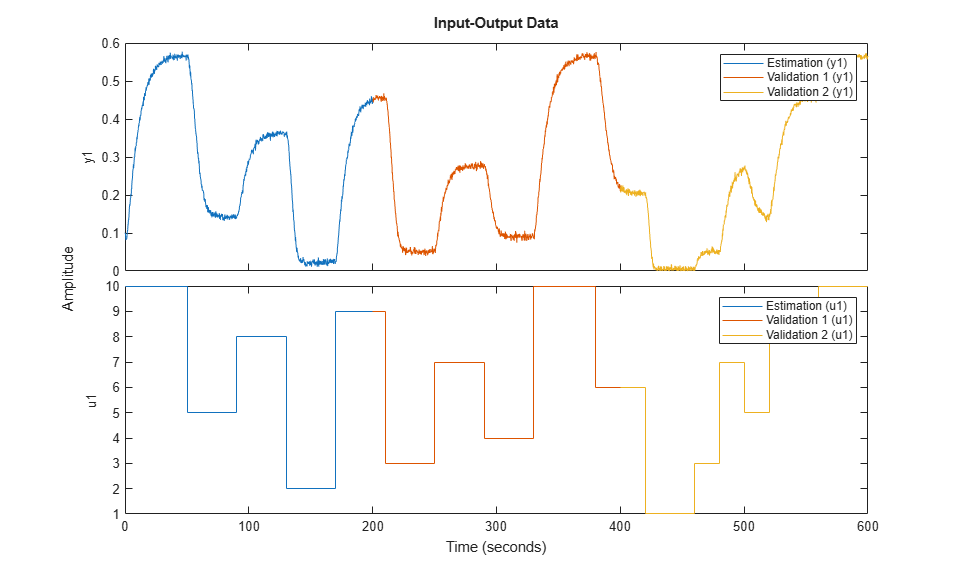### Regressor Selection

The first step in estimating nonlinear black box models is to pick the regressor set. The regressors are simple formulas based on time-delayed input/output variables, the simplest case being the variables lagged by a small set of consecutive values. For example, if "u" is the name of an input variable, and "y" the name of an output variable, then an example regressor set can be { y(t-1), y(t-2), u(t), u(t-1), u(t-2) }, where "t" denotes the time variable. Another example involving polynomial terms could be {abs(y(t-2)), y(t-2)*u(t-1), u(t-4)^2}. More complex, arbitrary formulas in the delayed variables are also possible.

In the System Identification Toolbox™, the regressors sets don't have to be created explicitly; a large collection can be generated implicitly by just specifying their form (such as polynomial order), the contributing variables and their lags. Specification objects `linearRegressor`, `polynomialRegressor` and `customRegressor` are used for this purpose. For example R = linearRegressor({'y','u'},{[1 2 4], [2 10]}) implies the regressor set {y(t-1), y(t-2), y(t-4), u(t-2), u(t-10)}. Similarly, R = polynomialRegressor({'y'},{[1 2]},3) implies 3rd order polynomial regressors in variable 'y' with lags 1 and 2, that is, the set {y(t-1)^3, y(t-2)^3}. More configuration choices are available such as using only the absolute values, and/or allowing mix of lags in the regressor formulas.

### Linear Regressor Specification Using an Order Matrix

When the model uses only linear regressors that are based on contiguous lags, it is often easier to specify them using an order matrix, rather than using a `linearRegressor` object. The order matrix [na nb nk], defines the numbers of past outputs, past inputs and the input delay used in the regressor formulas. This is the same idea that is used when estimating the linear ARX models (see ARX, IDPOLY). For example, NN = [2 3 4] implies that the output variable uses lags (1,2) and the input variable uses the lags (4,5,6) leading to the regressor set {y(t-1), y(t-2), u(t-4), u(t-5), u(t-6)}.

Usually model orders are chosen by trial and error. Sometimes it is useful to try linear ARX models first in order to get hints about the best orders. So let us first try to determine the optimal orders for a linear ARX model, using the functions `arxstruc` and `selstruc`.

```V = arxstruc(z1,z2,struc(1:5, 1:5,1:5)); % select best order by Akaike's information criterion (AIC) nn = selstruc(V,'aic') ```
```nn = 5 1 3 ```

The AIC criterion has selected nn = [na nb nk] = [5 1 3], meaning that, in the selected ARX model structure, y(t) is predicted by the 6 regressors y(t-1),y(t-1),...,y(t-5) and u(t-3). We will try to use these values when estimating nonlinear models.

### Nonlinear ARX (IDNLARX) Models

In an IDNLARX model, the model output is a nonlinear function of regressors, like y(t) = Fcn(y(t-1),y(t-1),...,y(t-5), u(t-3)).

IDNLARX models are typically estimated with the syntax:

```M = NLARX(Data, Orders, Fcn). ```

It is similar to the linear ARX command, with the additional argument "Fcn" specifying the type of nonlinear function. A Nonlinear ARX model produces its output by using a 2-stage transformation: 1. Map the training data (input-output signals) to a set of regressors. Numerically, the regressor set is a double matrix R, with one column of data for each regressor. 2. Map the regressors to a single output, y = Fcn®, where Fcn() is a scalar nonlinear function.

To estimate an IDNLARX model, after the choice of model orders, we need to pick the type of nonlinear mapping function Fcn() to use. Let us first try a Wavelet Network (idWaveletNetwork) function.

```mw1 = nlarx(z1,[5 1 3], idWaveletNetwork); ```

The Wavelet Network uses a combination of wavelet units to map the regressors to the output of the model. The model `mw1` is an @idnalrx object. It stores the nonlinear mapping function (idWaveletNetwork object here) in its `OutputFcn` property. The number of these units to use can be specified in advance, or we can leave it to the estimation algorithm to determine an optimal value automatically. The property NonlinearFcn.NumberOfUnits stores the chosen number of units in the model.

```NLFcn = mw1.OutputFcn; NLFcn.NonlinearFcn.NumberOfUnits ```
```ans = 3 ```

Examine the result by comparing the response of the model `mw1` to the measured output in the dataset `z1`.

```compare(z1,mw1); ```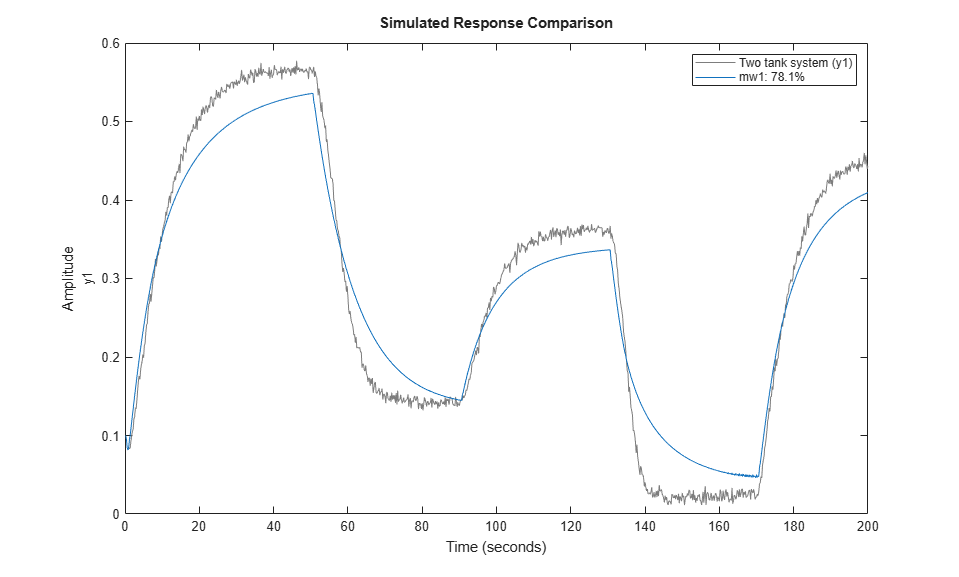### Using Model Properties

The first model was not quite satisfactory, so let us try to modify some properties of the model. Instead of letting the estimation algorithm automatically choose the number of units in the idWaveletNetwork function, this number can be explicitly specified.

```mw2 = nlarx(z1,[5 1 3], idWaveletNetwork(8)); ```

Default InputName and OutputName have been used.

```mw2.InputName mw2.OutputName ```
```ans = 1×1 cell array {'u1'} ans = 1×1 cell array {'y1'} ```

The regressors of the IDNLARX model can be viewed using the GETREG command. The regressors are made from `mw2.InputName`, `mw2.OutputName` and time delays.

```getreg(mw2) ```
```ans = 6×1 cell array {'y1(t-1)'} {'y1(t-2)'} {'y1(t-3)'} {'y1(t-4)'} {'y1(t-5)'} {'u1(t-3)'} ```

Note that the order matrix is equivalent to the linear regressor set created using the specification object, as in:

```R = linearRegressor([z1.OutputName; z1.InputName],{1:5, 3}); mw2_a = nlarx(z1, R, idWaveletNetwork(8)); getreg(mw2_a) ```
```ans = 6×1 cell array {'y1(t-1)'} {'y1(t-2)'} {'y1(t-3)'} {'y1(t-4)'} {'y1(t-5)'} {'u1(t-3)'} ```

`mw2_a` is essentially the same model as `mw2`. The use of a specification object allows more flexibility; use it when you do not want to use continguous lags, or have a minimum lag in output variables that is greater than one.

### Assigning Regressors to Mapping Functions

The output of an IDNLARX model is a static function of its regressors. Usually this mapping function has a linear block, a nonlinear block, plus a bias term (output offset). The model output is the sum of the outputs of these blocks and the bias. In order to reduce model complexity, only a subset of regressors can be chosen to enter the nonlinear block and the linear block. The assignment of the regressors to the linear and nonlinear components of the mapping function is performed using the RegressorUsage property of the model.

```RegUseTable = mw2.RegressorUsage ```
```RegUseTable = 6×2 table y1:LinearFcn y1:NonlinearFcn ____________ _______________ y1(t-1) true true y1(t-2) true true y1(t-3) true true y1(t-4) true true y1(t-5) true true u1(t-3) true true ```

RegUseTable is a table that shows which regressors are used as inputs to the linear and the nonlinear components of the Wavelet Network. Each row corresponds to one regressor. Suppose we want to use only those regressors that are functions of input variable 'u1' in the nonlinear component. This configuration can be achieved as follows.

```RegNames = RegUseTable.Properties.RowNames; I = contains(RegNames,'u1'); RegUseTable.("y1:NonlinearFcn")(~I) = false; ```

As an example, consider a model that uses polynomial regressors of order 2 in addition to the linear ones used by `mw2`. First, generate a polynomial regressor specification set.

```P = polynomialRegressor({'y1','u1'},{1:2, 0:3},2) ```
```P = <strong>Order 2 regressors in variables y1, u1</strong> Order: 2 Variables: {'y1' 'u1'} Lags: {[1 2] [0 1 2 3]} UseAbsolute: [0 0] AllowVariableMix: 0 AllowLagMix: 0 TimeVariable: 't' ```

Now add the specification `P` to the model's Regressors property.

```mw3 = mw2; % start with the mw2 structure for new estimation mw3.Regressors = [mw3.Regressors; P]; % add the polynomial set to the model structure ```

Finally, update the parameters of the model to fit the data by minimizing 1-step ahead prediction errors.

```mw3 = nlarx(z1, mw3) ```
```mw3 = <strong>Nonlinear ARX model with 1 output and 1 input</strong> Inputs: u1 Outputs: y1 Regressors: 1. Linear regressors in variables y1, u1 2. Order 2 regressors in variables y1, u1 Output function: Wavelet network with 8 units Sample time: 0.2 seconds Status: Estimated using NLARX on time domain data "Two tank system". Fit to estimation data: 96.76% (prediction focus) FPE: 3.499e-05, MSE: 3.338e-05 ```

### Evaluating Estimated Models

Different models, both linear and nonlinear, can be compared together in the same COMPARE command.

```mlin = arx(z1,[5 1 3]); % linear ARX model % compare(z1,mlin,mw2,mw3) ```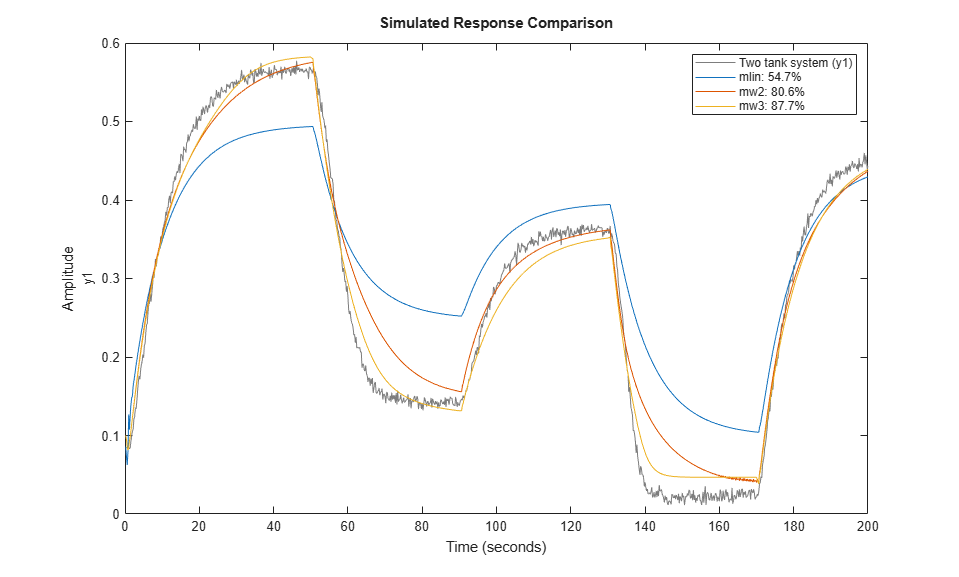Model validation can be carried out by running the COMPARE command on the validation datasets. First, validate the models by checking how well their simulated responses match the measured output signal in `z2`.

```compare(z2,mlin,mw2,mw3) ```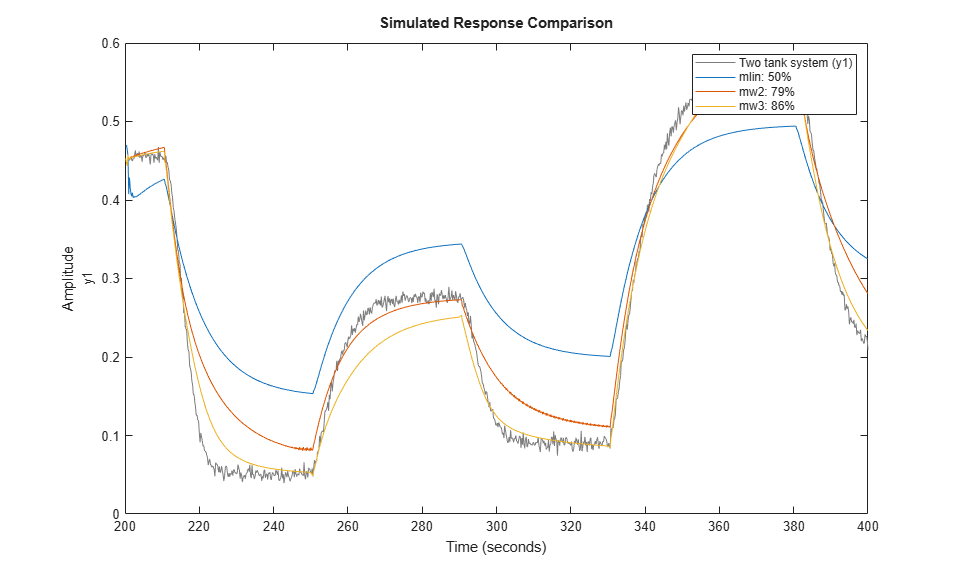Similarly, validate the estimated models against the dataset z3.

```compare(z3,mlin,mw2,mw3) ```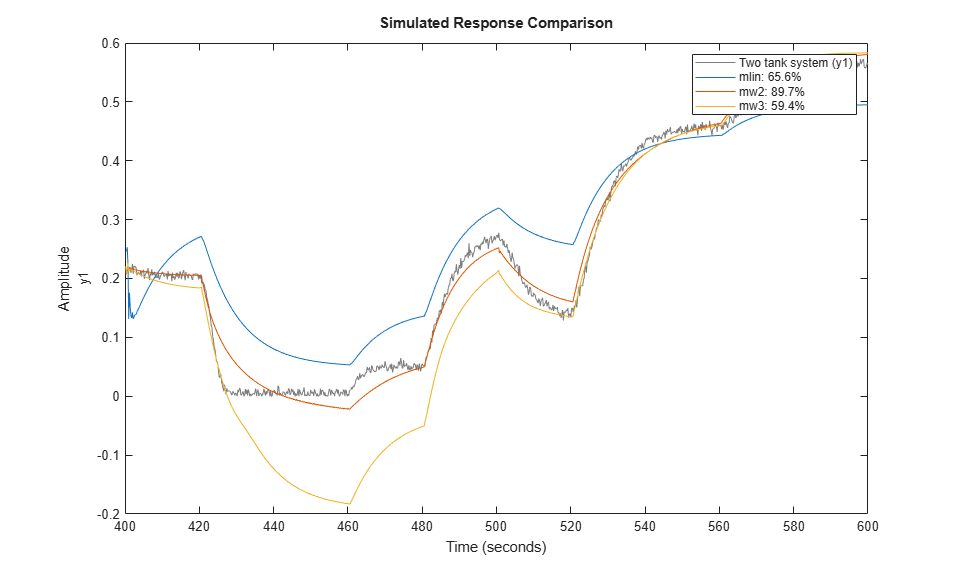An analysis of the model residues may also be performed to validate the model quality. We expect the residues to be white (uncorrelated with itself except at lag 0) and uncorrelated with the input signal. The resideus are the 1-step ahead prediction errors. The residues can be visualized with bounds on insignificant values by using the RESID command. For example on the valiation dataset `z2`, the residues for the three identified models are shown below.

```resid(z2,mlin,mw2,mw3) legend show ```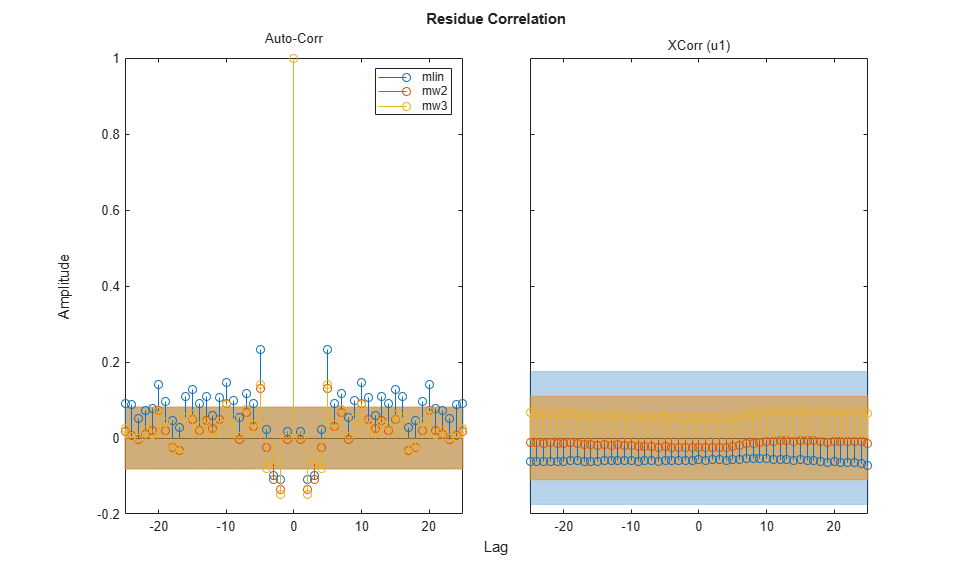The residues are all uncorrelated with the input signal of `z2` (z2.InputData). However, they exhibit some auto-correlation for the models `mlin` and `mw3`. The model `mw2` performs better on both (auto- and cross-correlation) tests.

Function PLOT may be used to view the nonlinearity responses of various IDNLARX models. The plot essentially shows the characteristics of the nonlinear mapping function Fcn().

```plot(mw2,mw3) % plot nonlinearity response as a function of selected regressors ```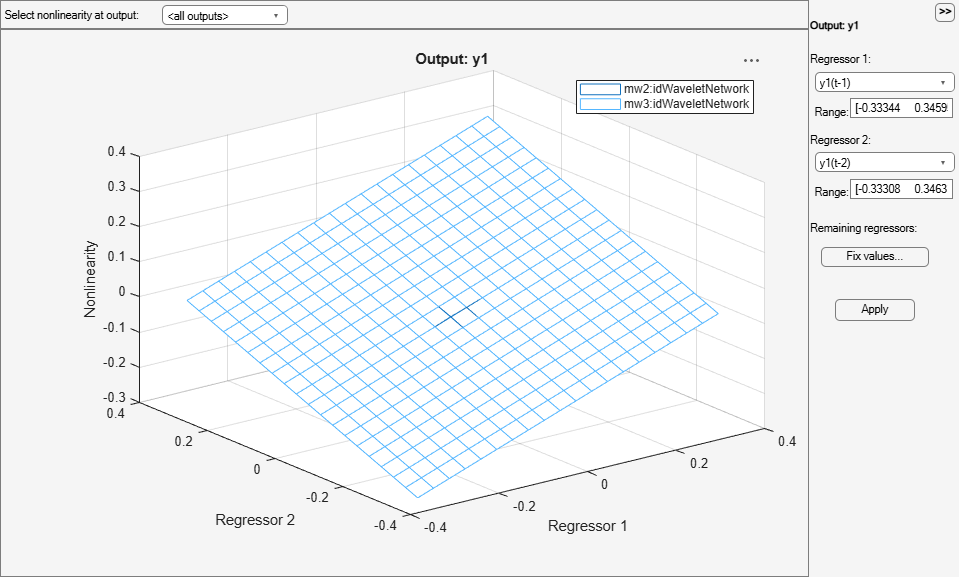### Nonlinear ARX Model with Sigmoid Network

In place of a Wavelet Network, other nonlinear functions can be used in the model. Try the idSigmoidNetwork function, which maps the regressors to the output using a sum of sigmoid units (plus a linear and an offset term). Configure the network to use 8 units of sigmoid (dilated and translated by different amounts).

```ms1 = nlarx(z1,[5 1 3], idSigmoidNetwork(8)); compare(z1,ms1) ```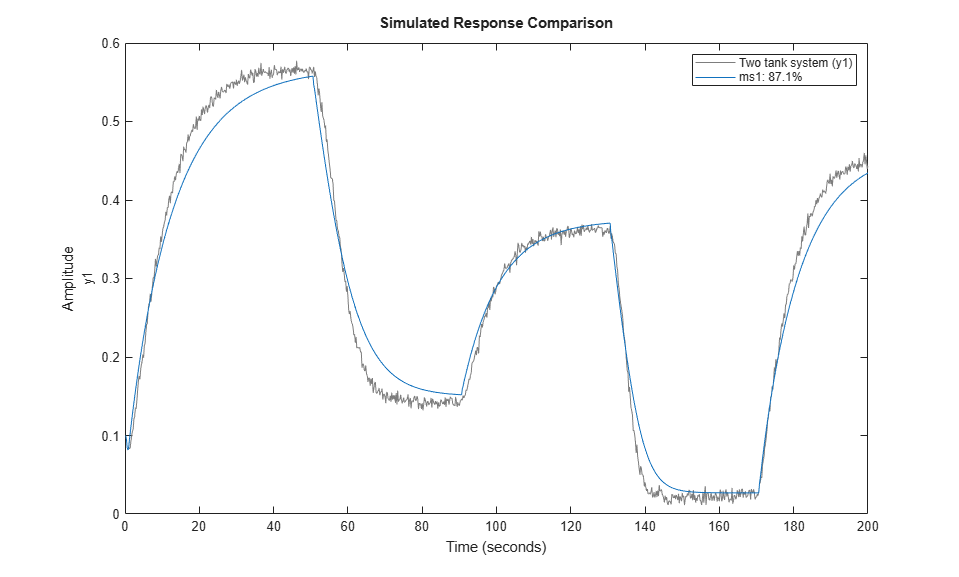Now evaluate the new model on the data set z2.

```compare(z2,ms1); ```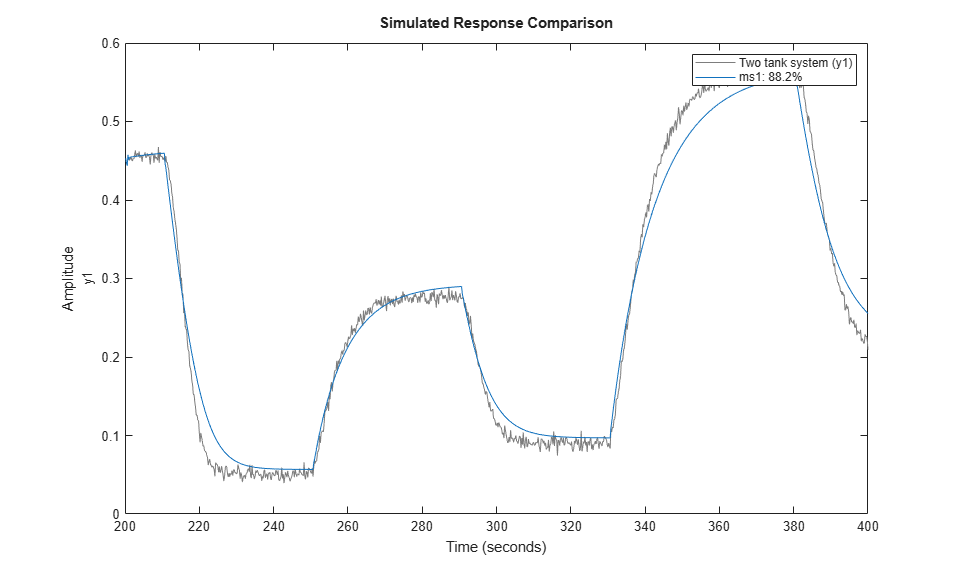and on the data set z3.

```compare(z3,ms1); ```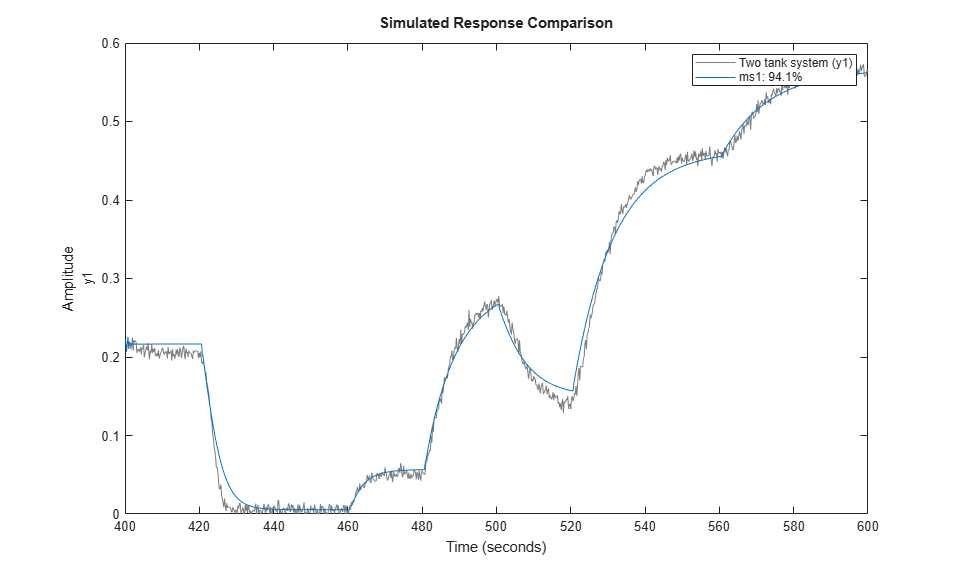### Other Nonlinear Mapping Functions in IDNLARX Model

idCustomNetwork is similar to idSigmoidNetwork, but instead of the sigmoid unit function, users can define their own unit function in MATLAB files. This example uses the gaussunit function.

```type gaussunit.m ```
```function [f, g, a] = gaussunit(x) %GAUSSUNIT Unit function for use in idCustomNetwork (example) % %[f, g, a] = GAUSSUNIT(x) % % x: unit function variable % f: unit function value % g: df/dx % a: unit active range (g(x) is significantly non zero in the interval [-a a]) % % The unit function must be "vectorized": for a vector or matrix x, the output % arguments f and g must have the same size as x, computed element-by-element. % Copyright 2005-2021 The MathWorks, Inc. % Author(s): Qinghua Zhang f = exp(-x.*x); if nargout>1 g = - 2*x .* f; a = 0.2; end ```

For the customnet based model, use only the third, fifth and the sixth regressors as input to the nonlinear component of the customnet function.

```mc1_ini = idnlarx('y1','u1', [5 1 3], idCustomNetwork(@gaussunit)); Use = false(6,1); Use([3 5 6]) = true; mc1_ini.RegressorUsage{:,2} = Use; mc1 = nlarx(z1,mc1_ini); ```

Tree partition function is another mapping function that can be used in the Nonlinear ARX model. Estimate a model using idTreePartition function:

```mt1 = nlarx(z1, [5 1 3], idTreePartition); ```

Compare responses of models `mc1` and `mt1` to data `z1`.

```compare(z1, mc1, mt1) ```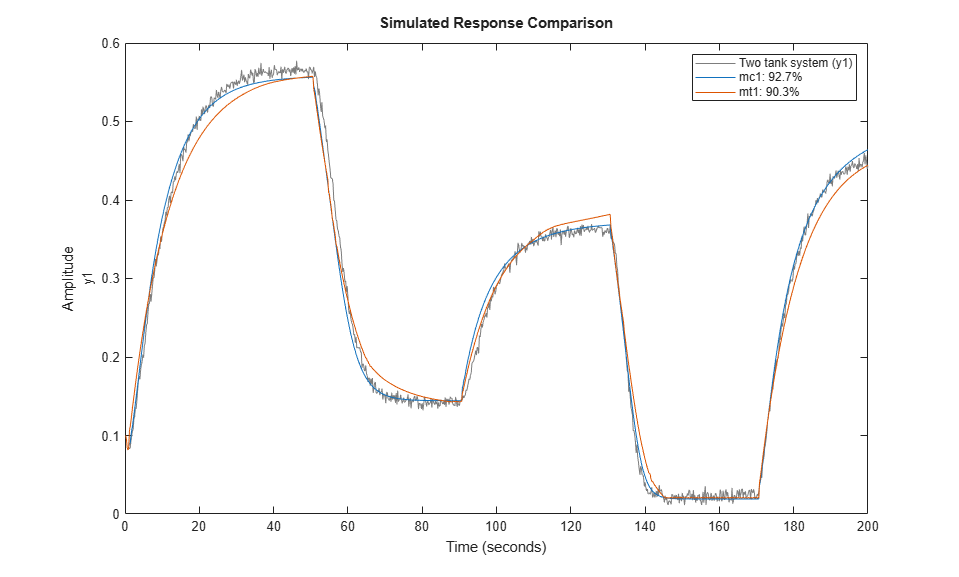### Using Regression Algorithms from Statistics and Machine Learning Toolbox

If you have access to the Statistics and Machine Learning Toolbox™ you can also use gaussian process (GP) functions and bagged or boosted tree ensembles to create your Nonlinear ARX models. The GPs are represented by the idGaussianProcess object, while regression tree ensembles are represented by the idTreeEnsemble object. GPs use squared-exponential kernel by default. Other kernel types may be specified when creating the object. In the example here, we use a 'Matern32' kernel.

```if exist('fitrgp','file')==2 Warn = warning('off','stats:lasso:MaxIterReached'); mp1 = nlarx(z1, [5 1 3], idGaussianProcess('Matern32')) % Create boosted ensemble of 200 regression trees En = idTreeEnsemble; En.EstimationOptions.FitMethod = 'lsboost-resampled'; En.EstimationOptions.NumLearningCycles = 200; En.EstimationOptions.ResampleFraction = 1; mp2 = nlarx(z1, [0 70 3], En) compare(z1, mp1, mp2) % compare model fits to estimation data warning(Warn) end ```
```mp1 = <strong>Nonlinear ARX model with 1 output and 1 input</strong> Inputs: u1 Outputs: y1 Regressors: Linear regressors in variables y1, u1 Output function: Gaussian process function using a Matern32 kernel. Sample time: 0.2 seconds Status: Estimated using NLARX on time domain data "Two tank system". Fit to estimation data: 97.1% (prediction focus) FPE: 2.738e-05, MSE: 2.675e-05 mp2 = <strong>Nonlinear ARX model with 1 output and 1 input</strong> Inputs: u1 Outputs: y1 Regressors: Linear regressors in variables u1 Output function: Bagged Regression Tree Ensemble Sample time: 0.2 seconds Status: Estimated using NLARX on time domain data "Two tank system". Fit to estimation data: 91.14% MSE: 0.0002503 ```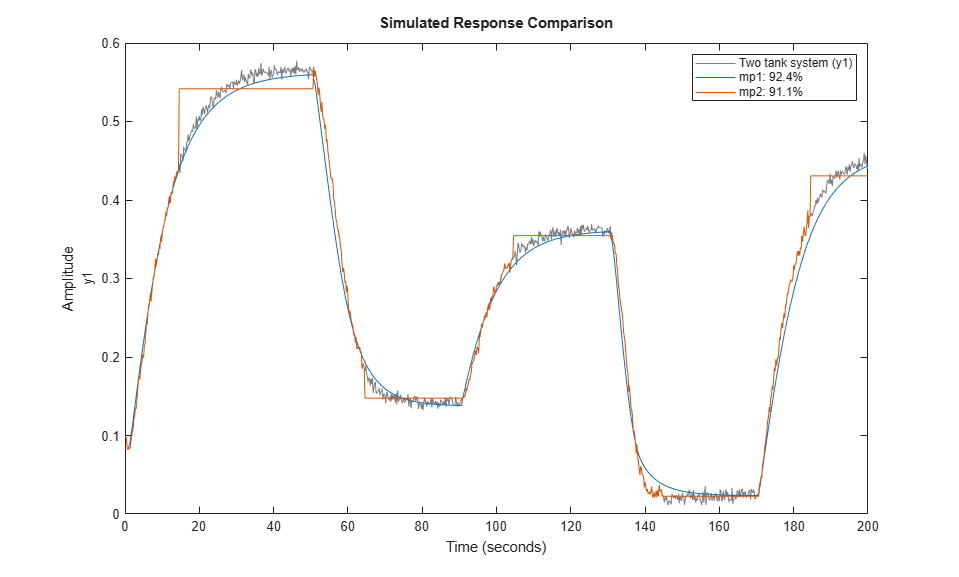### Using the Network Object from Deep Learning Toolbox

If you have the Deep Learning Toolbox™ available, you can also use a neural network to define the mapping function Fcn() of your IDNLARX model. This network must be a feed-forward network (no recurrent component).

Here, we will create a single-output network with an unknown number of inputs (denote by input size of zero in the network object). The number of inputs is set to the number of regressors during the estimation process automatically.

```if exist('feedforwardnet','file')==2 % run only if Deep Learning Toolbox is available ff = feedforwardnet([5 20]); % use a feed-forward network to map regressors to output ff.layers{2}.transferFcn = 'radbas'; ff.trainParam.epochs = 50; % Create a neural network mapping function that wraps the feed-forward network OutputFcn = idFeedforwardNetwork(ff); mn1 = nlarx(z1,[5 1 3], OutputFcn); % estimate network parameters compare(z1, mn1) % compare fit to estimation data end ```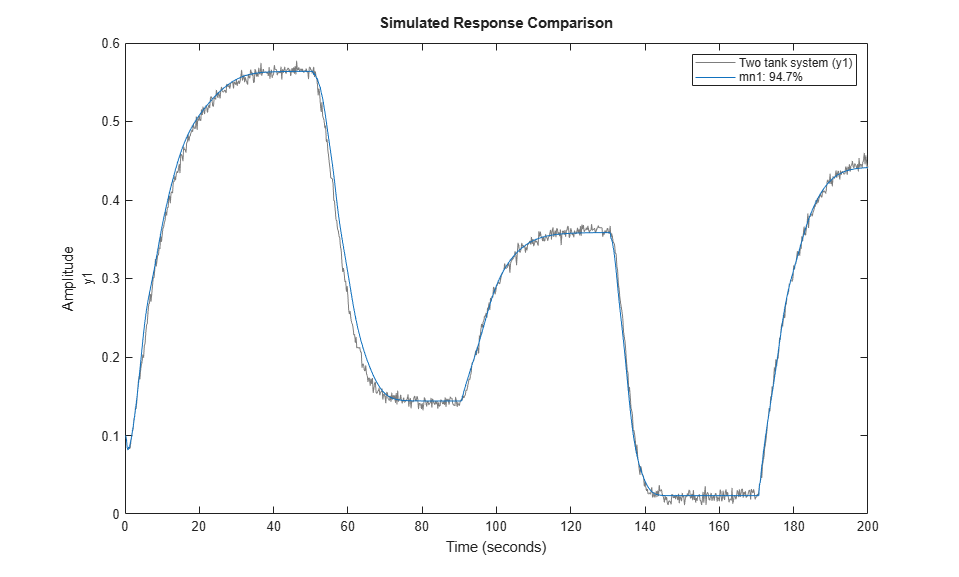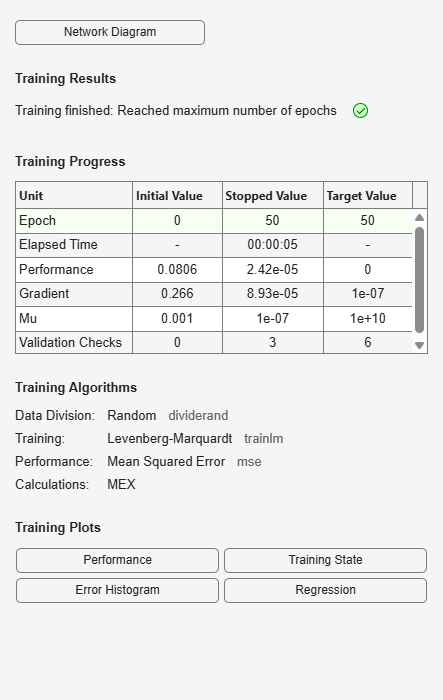### Hammerstein-Wiener (IDNLHW) Models

A Hammerstein-Wiener model is composed of up to 3 blocks: a linear transfer function block is preceded by a nonlinear static block and/or followed by another nonlinear static block. It is called a Wiener model if the first nonlinear static block is absent, and a Hammerstein model if the second nonlinear static block is absent.

IDNLHW models are typically estimated with the syntax:

```M = NLHW(Data, Orders, InputNonlinearity, OutputNonlinearity). ```

where Orders = [nb bf nk] specifies the orders and delay of the linear transfer function. InputNonlinearity and OutputNonlinearity specify the nonlinear functions for the two nonlinear blocks, at the input and output end of the linear block. M is an @idnlhw model. The linear Output-Error (OE) model corresponds to the case of trivial (unit gain) nonlinearities, that is, if the input and the output nonlinear functions of an IDNLHW model are both unit gains, the model structure is equivalent to a linear OE model.

### Hammerstein-Wiener Model with the Piecewise Linear Nonlinear Function

The idPiecewiseLinear (piecewise linear) nonlinear function is useful in general IDNLHW models.

Notice that, in Orders = [nb nf nk], nf specifies the number of poles of the linear transfer function, somewhat related to the "na" order of the IDNLARX model. "nb" is related to the number if zeros.

```mhw1 = nlhw(z1, [1 5 3], idPiecewiseLinear, idPiecewiseLinear); ```

The result can be evaluated with COMPARE as before.

```compare(z1,mhw1) ```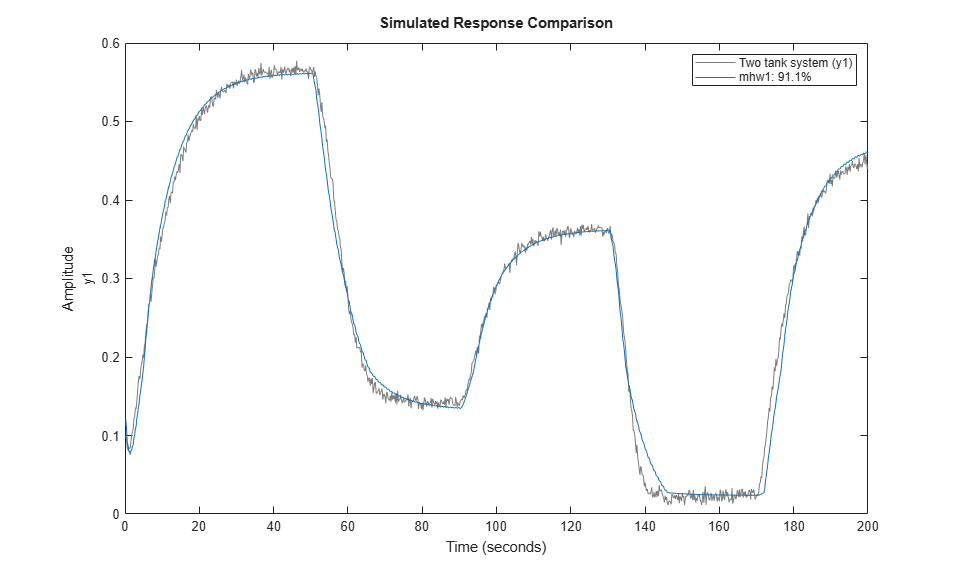Model properties can be visualized by the PLOT command.

Click on the block-diagram to choose the view of the input nonlinearity (UNL), the linear block or the output nonlinearity (YNL).

For the linear block, it is possible to choose the type of plots within Step response, Bode plot, Impulse response and Pole-zero map.

```plot(mhw1) ```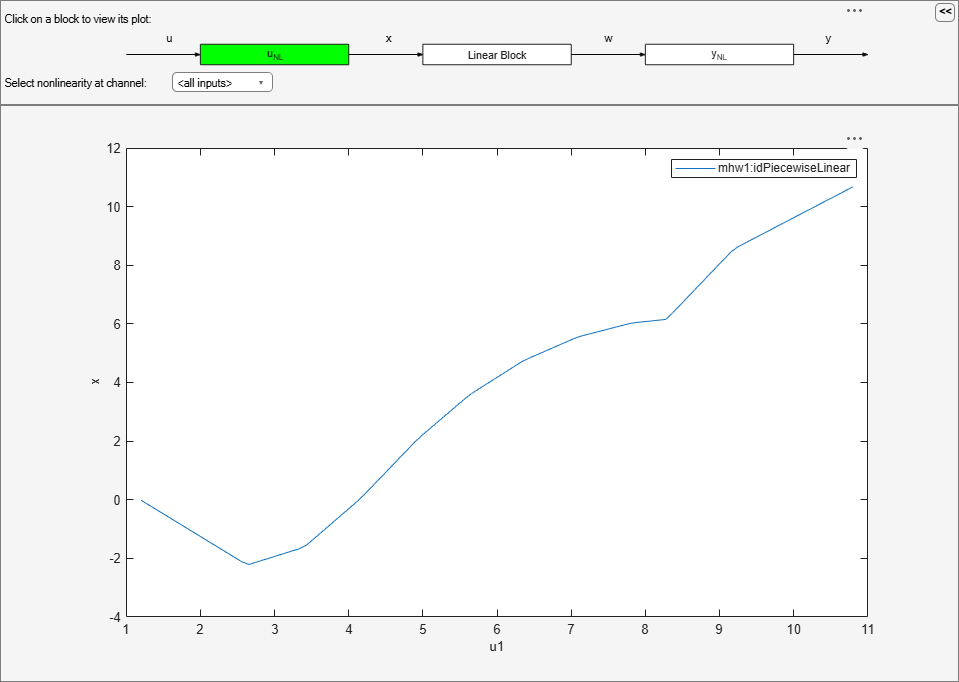The break points of the two piecewise linear functions can be examined. This is a two row matrix, the first row corresponds to the input and the second row to the output of the idPiecewiseLinear function.

```mhw1.InputNonlinearity.BreakPoints ```
```ans = Columns 1 through 7 2.6795 3.4262 4.1729 4.9195 5.6324 6.3599 7.0874 -2.0650 -1.6316 -0.2396 1.4602 2.7554 3.6889 4.2632 Columns 8 through 10 7.8148 8.3543 9.1260 4.5074 4.4653 6.2634 ```

### Hammerstein-Wiener Model with Saturation and Dead Zone Nonlinearities

The saturation and dead-zone functions are physics inspired nonlinear functions. They can be used to describe actuator/sensor saturation scenario and dry friction effects.

```mhw2 = nlhw(z1, [1 5 3], idDeadZone, idSaturation); compare(z1,mhw2) ```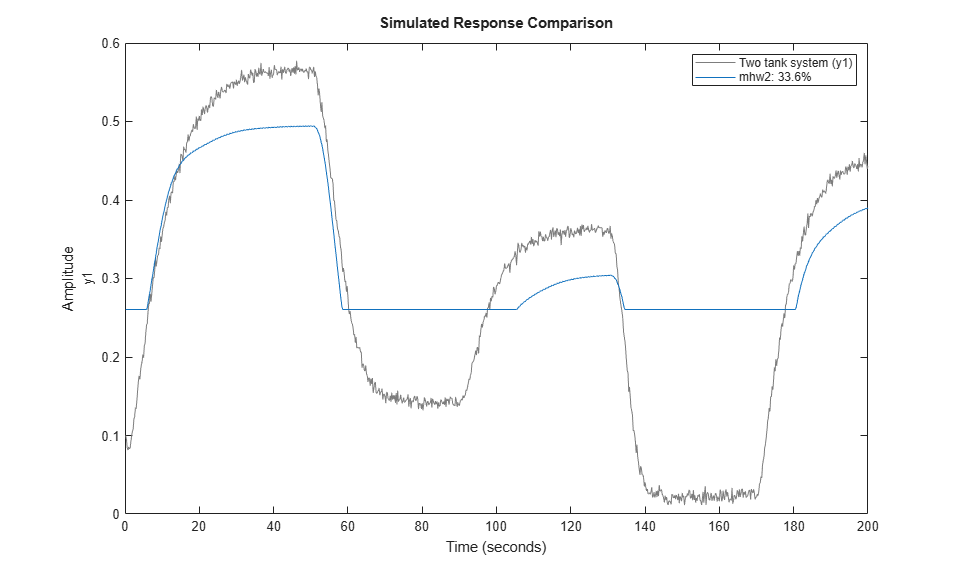The absence of a nonlinearity is indicated by the IDUNITGAIN object, or by the empty "[]" value. A unit gain is a just a feed-through of an input signal to the output without any modification.

```mhw3 = nlhw(z1, [1 5 3], idDeadZone, idUnitGain); % no output nonlinearity mhw4 = nlhw(z1, [1 5 3], [],idSaturation); % no input nonlinearity ```

The limit values of dead-zone and saturation functions can be examined after estimation.

```mhw2.InputNonlinearity.ZeroInterval mhw2.OutputNonlinearity.LinearInterval ```
```ans = -0.9982 4.7565 ans = 0.2601 0.5848 ```

The initial guess of ZeroInterval for dead-zone or LinearInterval for saturation function can be specified while estimating the model.

```mhw5 = nlhw(z1, [1 5 3], idDeadZone([0.1 0.2]), idSaturation([-1 1])); ```

### Hammerstein-Wiener Model - Specifying Properties

The estimation algorithm options can be specified using the NLHWOPTIONS command.

```opt = nlhwOptions(); opt.SearchMethod = 'gna'; opt.SearchOptions.MaxIterations = 7; mhw6 = nlhw(z1, [1 5 3], idDeadZone, idSaturation, opt); ```

An already-estimated model can be refined by more estimation iterations.

Evaluated on the estimation data `z1`, `mhw7` should have a better fit than `mhw6`.

```opt.SearchOptions.MaxIterations = 30; mhw7 = nlhw(z1, mhw6, opt); compare(z1, mhw6, mhw7) ```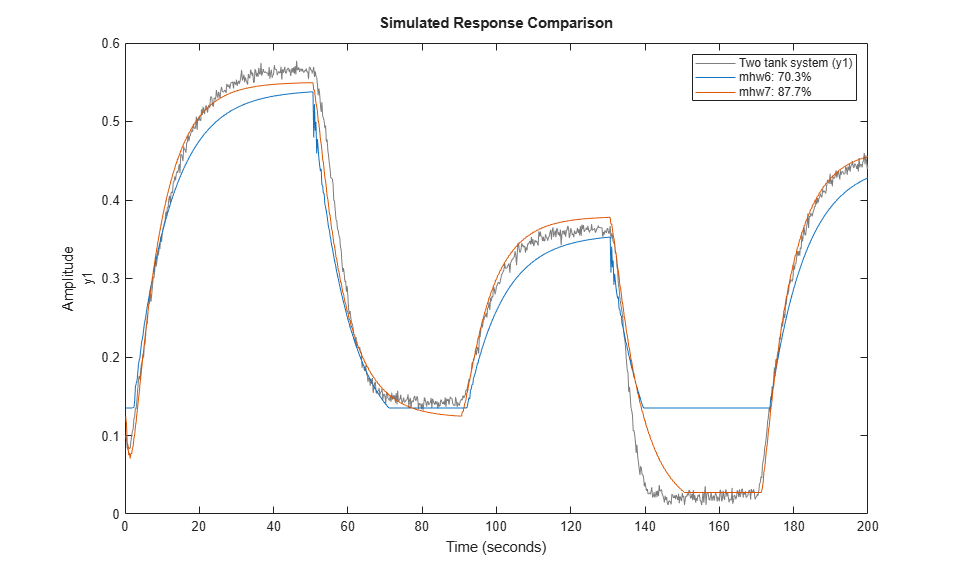### Hammerstein-Wiener Model - Use Other Nonlinear Functions

The nonlinear functions Piecewise Linear (idPiecewiseLinear), Saturation (idSaturation) and Dead Zone (idDeadZone) have been mainly designed for use in IDNLHW models. They can only estimate single variable nonlinearities. The other more general nonlinear functions, Sigmoid Network (idSigmoidNetwork), Custom Network (idCustomNetwork) and Wavelet Network (idWaveletNetwork) can also be used in IDNLHW models (besides the IDNLARX models). However, the non-differentiable functions Tree-partition (idTreePartition), Multi-layer Neural Network (idFeedforwardNetwork), Gaussian Process functions (idGaussianProcess) and Regression Tree Ensembles (idTreeEnsemble) cannot be used because the estimation of IDNLHW models require differentiable nonlinear functions.